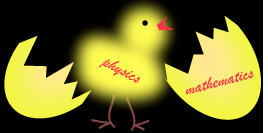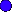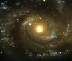Which Came First, Mathematics or Physics?
Chicken-Egg-Chicken-Egg......?
Physics is the fundamental study of Nature, in other words, in physics we want to find out how stuff works. Mathematics, on the other hand, is harder to pin down since it exists only in the human mind. Mathematics epitomizes the word "abstract." But still this can't be the complete story because many mathematical structures can be used to describe how physical objects interact and the nature of the relationships among them.

In physics we use mathematics as a tool to understand Nature. In mathematics, the pure notions of numbers and other structures do not need physics to exist or explain or even justify them. But the surprising thing is that often some newly discovered abstract formulation in mathematics turns out, years later, to describe physical phenomena which we hadn't known about earlier. When, as a student of physics, you see this for the first time, it is truly overwhelming. The only conclusion I can bring myself to is that mathematics is not just a tool of physics; it must be much, much more. Since mathematics is a product of our imagination, then somehow the structure of the universe itself seems to be imprinted on the human mind. And if that is the case, the relationship between mathematics and physics does indeed boil down to the chicken-and-the-egg question.

A One Way Street
It is important that I emphasize here that physics cannot be derived from mathematics alone. Let me back up a bit and fill in the holes. In order to understand fully a physical process, physicists try to derive the process from other more fundamental concepts. For example, in the early 1600's Johannes Kepler constructed a model of the solar system which he then used to predict the exact locations of planets with hitherto unheard-of precision. This is usually the first step in trying to understand a physical system--figure out HOW it works and then try to figure out WHY it works that way. It took Sir Isaac Newton's formulation of gravity to explain why Kepler's model works. And it took Newton's discovery of three fundamental ways that matter interacts to derive his theory of gravity. So, in the end, starting with Newton's three "rules," you can derive Kepler's model: The planets move the way they do because of gravity, and gravity works the way it does because it follows three basic rules or "laws" for forces. This is what we mean by deriving a complicated physical concept from more simple ones.

But if you start from basic mathematical concepts alone, it is not possible to derive the existence of gravity or any of Newton's laws. Doesn't it seem to you that something strange is going on here? It's as if you can go from A to B but not from B to A, and we're left to wander around in a one-way street.

Here are some discussion questions you might think about ==> ?

Let's see.....
I would like to show you some concrete examples of pure mathematics (that came right out of the imagination of mathematicians) which no one at the time thought had any uses in physics. Yet later on, the abstract mathematics turned out to describe physical concepts not dreamed of earlier. The mathematical scaffolding was already in place.

The following stories will require some concentration on your part, but if you stick with it, you will come away with a whole new view on how mathematics and physics are intertwined. The first two examples show how some abstract mathematical ideas preceded the physics they ended up describing. The third example shows the reverse: a physical concept that forced mathematical progress (a much rarer thing!).The first example is about Einstein's General Theory of Relativity.The second example concerns the how Imaginary Numbers gave us computers!The third example tells the story of how Newton invented a new math because he needed a way to describe a physical process.